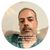# New to Qlik Sense

If you’re new to Qlik Sense, start with this Discussion Board and get up-to-speed quickly.

Announcements
Join us for a live Q&A! September 21, 10 AM ET - Onboarding Fast in Qlik Sense SaaS! REGISTER
cancel
Showing results for
Did you mean:Creator

## I have a scenario like language translation

I have two tables, in which one table contains list of languages, other table contains the translation information, according the user selection language the dashboard will translate to that language.

there is no relation between two tables.

How can i we do this, can any one help me in this.

I'm attaching the QVD files.

4 RepliesPartner

You can can refer to this in which I've talked about such a thing (translation):

Creating our own Narratives in Sense by Omar BEN SALEM. | LinkedIn

In fact, I was enjoying using the Qlik Sense Narrative extension till It became no free no more.. For those of you not familiar with the Narrative extension; here's a link to it :

Basically, it describes a chart in a humain language.

Why? Because sometimes, even though a graphic chart could be very powerful to analyze data, some users always prefer a good old humain way summary.

With that in mind, I thought about creating my own version of a chart's summary:Today, we'll be describing how to achieve such a thing.

Ps: Even though the expressions used can appear complex; It's all generic expressions; you'll just have to change the dimension and measure to replicate all the expressions based on your own data.

First of all, let's build a test application everybody can use; to do so: go to the script and tap : ctrl+00 and Qlik Sense will automatically generate a script we can use in our test:Now, let's create the Chart we want to summarize:

• Create a bar chart
• As a dimension : =Num&' : ('& num(aggr((Sum(Expression1)/sum(total Expression1)),Num),'# ##0,00%')&')'• As a measure: Sum(Expression1)
• Color by expression as follow to have the Num (dimension) with the highest sum(Expression1) (Measure) colored in green, the lowest in red and the other in grey:

if(Num= '\$(=FirstSortedValue(Num,- aggr(sum(Expression1),Num)))',Green(),if(Num= '\$(=FirstSortedValue(Num, aggr(sum(Expression1),Num)))',red(), '#7b7a78'))Now, let's create a text and image chart to describe the main points related in this bar chart :We'll be showing :

• the sum(Expression1) for the selected period
• the average for the selected period
• the dimension with highest and lowest measures for the selected period and the difference btwn them
• the difference between the left and right borns.

To even make our narrative better, we'll be creating a variable : vLanguage and 2 buttons objects (using this certified extension https://github.com/stefanwalther/sense-navigation ).

First button to show the narrative in English (vLangage=1) / Second button to show narrative in French (vLanguage=2)

Let's begin :

1) First thing to do is creating a title for our text and image object :

=if(vLangue=1,'A narrative of the main points relative to the bar chart :', 'Ci-dessous un résumé des informations clés récoltées du graphique en bars')2) Create our measures:

Measure1: =if(vLangue=1,'* In the selected frame, ' , '* Dans la partie sélectionnée, ' )

Measure2: =if(vLangue=1,'FIRST is number '& FirstSortedValue(Num,- aggr(sum(Expression1),Num)), 'le 1er est '& FirstSortedValue(Num,- aggr(sum(Expression1),Num)))

Measure3: =if(vLangue=1,' with '&num(sum({<Num={"\$(= FirstSortedValue(Num,- aggr(sum(Expression1),Num)))"}>} Expression1),'# ##0')&' points ',' avec '&num(sum({<Num={"\$(= FirstSortedValue(Num,- aggr(sum(Expression1),Num)))"}>} Expression1),'# ##0')&' points ')

Measure4: =if(vLangue=1,', the LAST is number '&FirstSortedValue(Num, aggr(sum(Expression1),Num)), ', le dernier est '&FirstSortedValue(Num, aggr(sum(Expression1),Num)))

Measure5: =if(vLangue=1,' with '&num(sum({<Num={"\$(= FirstSortedValue(Num, aggr(sum(Expression1),Num)))"}>} Expression1),'# ##0'), ' avec '&num(sum({<Num={"\$(= FirstSortedValue(Num, aggr(sum(Expression1),Num)))"}>} Expression1),'# ##0'))

Measure6: =if(vLangue=1, '* The Sum is : ','* La somme est de : ')

Measure7: num(sum(Expression1),'# ##0')&' points'

Measure8: =if(vLangue=1, '* The Average is : ','* La moyenne est de : ')

Measure9: =num((sum(Expression1) / count(distinct Num)),'# ##0,00')&' points'

Measure10: =if(vLangue=1,'* The difference between the FIRST ('& FirstSortedValue(Num,- aggr(sum(Expression1),Num))&')'&' and the last ('&FirstSortedValue(Num, aggr(sum(Expression1),Num))&') is : ', '* La différence entre le 1er ('& FirstSortedValue(Num,- aggr(sum(Expression1),Num))&')'&' et le dernier '&FirstSortedValue(Num, aggr(sum(Expression1),Num))&') est : ')

Measure11: =if(vLangue=1,num((sum({<Num={"\$(= FirstSortedValue(Num,- aggr(sum(Expression1),Num)))"}>} Expression1) -sum({<Num={"\$(= FirstSortedValue(Num, aggr(sum(Expression1),Num)))"}>} Expression1)),'# ##0')& ' points', num((sum({<Num={"\$(= FirstSortedValue(Num,- aggr(sum(Expression1),Num)))"}>} Expression1) -sum({<Num={"\$(= FirstSortedValue(Num, aggr(sum(Expression1),Num)))"}>} Expression1)),'# ##0')& ' points')

Measure12: =if(vLangue=1,'* The difference between the right born ('&max(Num)&') '&'and the left one('& min(Num)&')'&' is : ', '* La différence entre la barre droite ('&max(Num)&') '&'et gauche('& min(Num)&')'&' est : ')

Measure13: =if(vLangue=1,num((sum({<Num={"\$(=max(Num))"}>}Expression1) - sum({<Num={"\$(=min(Num))"}>}Expression1)),'# ##0')&' points', num((sum({<Num={"\$(=max(Num))"}>}Expression1) - sum({<Num={"\$(=min(Num))"}>}Expression1)),'# ##0')&' points')

Now make sure you depose the measures the right way:Result:if we select Français, It'll be translated in French:If we select another frame of values in our chart, the narrative will automatically focus on the newly selected values:Hope you like it !MVP

You may use cross table to make relation like this -

Translation:

"Index",

English,

Japanese,

French,

German,

Portuguese,

Spanish,

Bulgarian

FROM [lib://source/Translations.qvd]

(qvd);

Crosstable(Language,Text)

resident Translation;

Drop table Translation;

Language:

_LANGUAGE as Language

FROM [lib://source/LanguageList.qvd]

(qvd);Specialist

Somehow I am not being able to see  Language list in fields when it is in data.Specialist

How to remove the hidden Tag?Tags
Community Browser